# CS 294: Fairness in Machine Learning

## Moritz HardtAugust 25, 2017

## Logistics

This is a CS grad seminar. Prereqs: Stats, ML, probability, linear algebra

Web site: fairmlclass.github.io

Online dicussions on Slack:

• TODO: Email me for slack access link.
• You may join anonymously (won't affect grade).

Grade: 50% in-class participation, 50% project

Not yet enrolled? Talk to me after class today.## Rough course outline

Part I: Sources of unfairness

Part II: Observational fairness criteria

Part III: Beyond observational fairness criteria

Part IV: Measurement and sampling

Part V: Legal and policy perspectives

## Goal for this class

Develop a better understanding of a complex social problem that will allow us to contribute to a meaningful technical discussion.

## The dangers of math snobbery

• Technical work without understanding social context.
• Thinking we're more rigorous than social scientists.
• Justifying an approach by the math it entails.

## Sanity checks for taking this class

You don't mind reading social science papers

You'd still be here even if the chance of getting a paper out of it is 0.

## Part I: Sources of unfairness

How can machine learning wind up
being unfair without any explicit wrongdoing?

## The sample size disparityGenerally, more data means smaller error

By definition, less data on minority groups.

Can lead to higher error rates on minority.

## The meaning of low error

Two classifiers with 5% average error:## Biases in data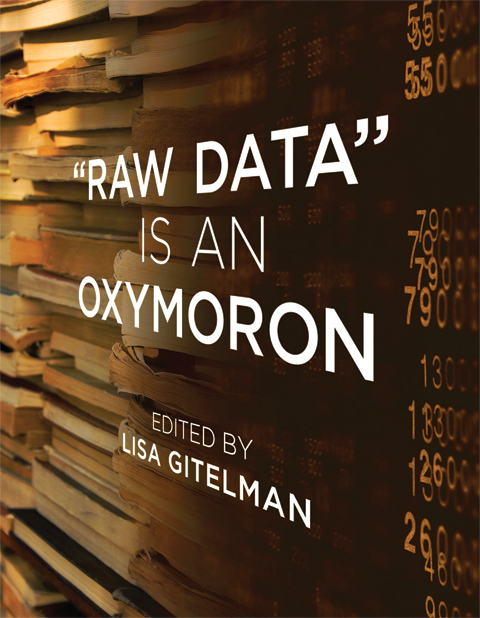## Biases in data

Collection:

• Demographic, geographic, behavioral, temporal biases,

Measurement:

• What do we choose to measure? How do we measure (e.g., grit)?

Pre-existing biases

• gender roles in text and images, racial stereotypes in language

## ML models reflect underlying data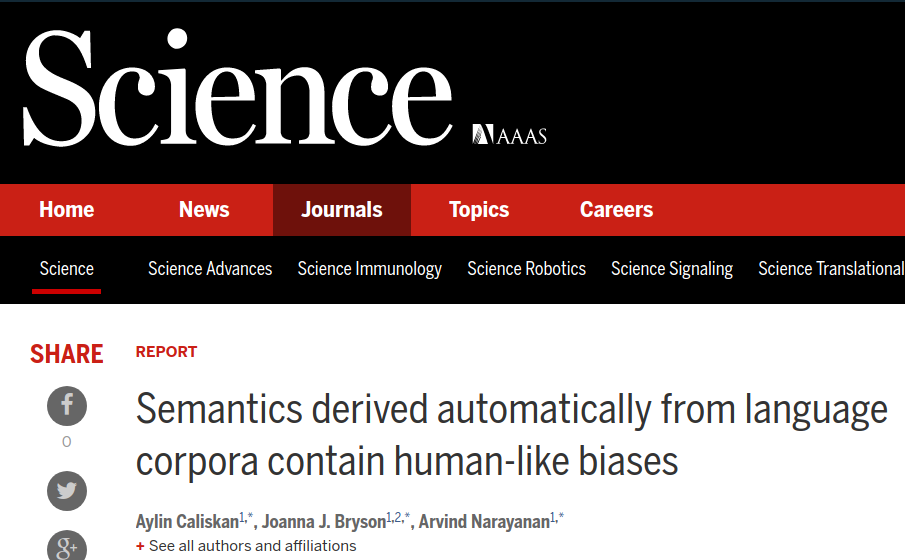## ML models reflect underlying data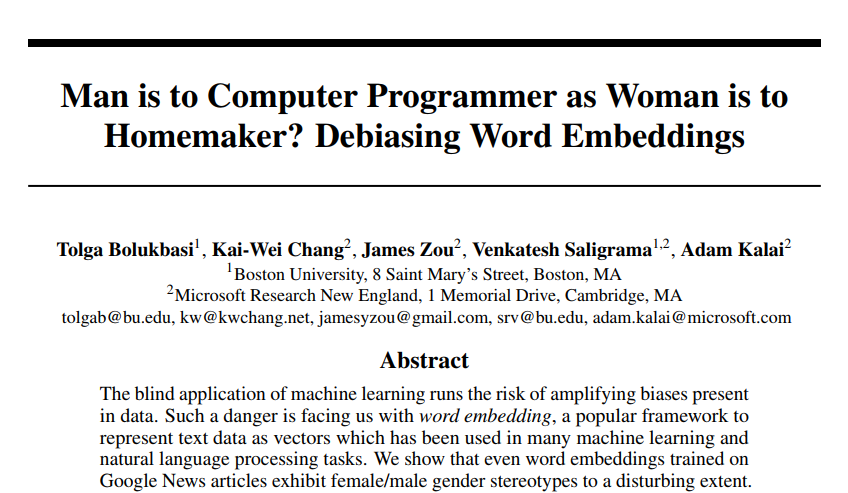## A sequence of White House reports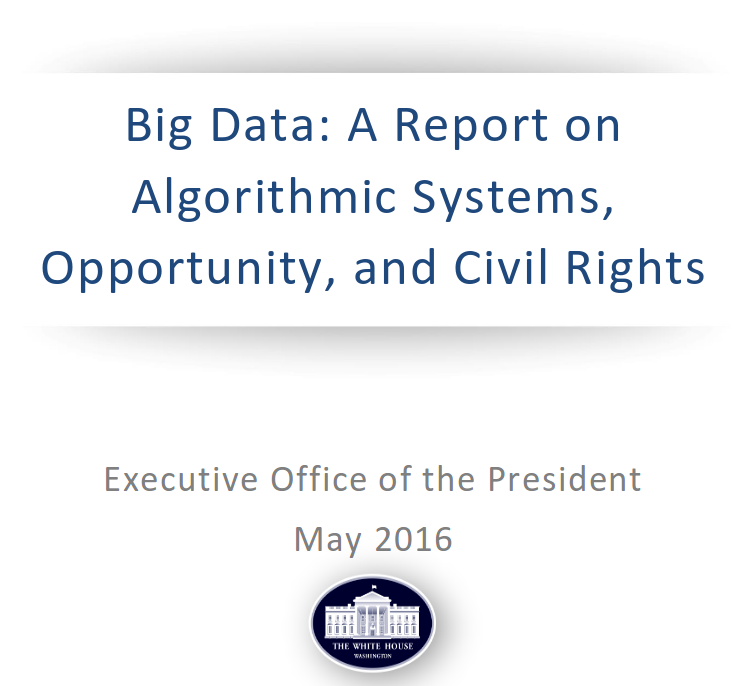## On Monday

We'll discuss:

Barocas, Selbst. Big Data's Disparate Impact

Before we meet:

Skim the whole thing. Choose one part to read very carefully.

• Boyd and Crawford, “Critical Questions for Big Data”
• O'Neill, Weapons of Math Destruction
• Pasquale, The Black Box Society
• The White House Office of Science and Technology Policy, Big Data: A Report on Algorithmic Systems, Opportunity, and Civil Rights

## Part II:Observational fairness criteria

Many definitions

Algorithms for achieving them

Impossibility results

## Typical setup

$X$ features of an individual

$A$ sensitive attribute (race, gender, ...)

$C=C(X,A)$ classifier mapping $X$ and $A$ to some prediction

$Y$ actual outcome

Note: random variables in the same probability space

## All of this is a lie

$X$ incorporates all sorts of measurement biases

$A$ often not even known, ill-defined, misreported, inferred

$C$ often not well defined, e.g., large production ML system

$Y$ often poor proxy of actual variable of interest

## Demographic parity

Assume $C$ and $A$ are binary $0/1$-variables.

Definition. Classifier $C$ satisfies demographic parity if
$\mathbb{P}\{ C = 1 \mid A = 1 \} = \mathbb{P}\{ C = 1 \mid A = 0 \}$.

## Accuracy parity

Assume $A$ is binary $0/1$-variable.

Definition. Classifier $C$ satisfies accuracy parity if
$\mathbb{P}\{ C = Y \mid A = 1 \} = \mathbb{P}\{ C = Y \mid A = 0 \}$.

## Precision parity

Assume $C$, $Y$ and $A$ are binary $0/1$-variables.

Definition. Classifier $C$ satisfies precision parity if
$\mathbb{P}\{ Y = 1 \mid C=1, A = 1 \} = \mathbb{P}\{ Y = 1\mid C=1, A = 0 \}$.

## True positive parity

Assume $C$, $Y$ and $A$ are binary $0/1$-variables.

Definition. Classifier $C$ satisfies true positive parity if
$\mathbb{P}\{ C = 1 \mid Y=1, A = 1 \} = \mathbb{P}\{ C = 1\mid Y=1, A = 0 \}$.

## Observational criteria

Definition. A criterion is called observational if it is a property of the joint distribution of features $X,A$, classifier $C$, and outcome $Y$.

Examples: Everything we just saw, and many others.

## Questions we'll work on

What can we learn from observational criteria?

How can we achieve them algorithmically?

How do these criteria shape public discourses?

Key example: COMPAS debate on crime recidivism risk scores

## COMPAS: An observational debate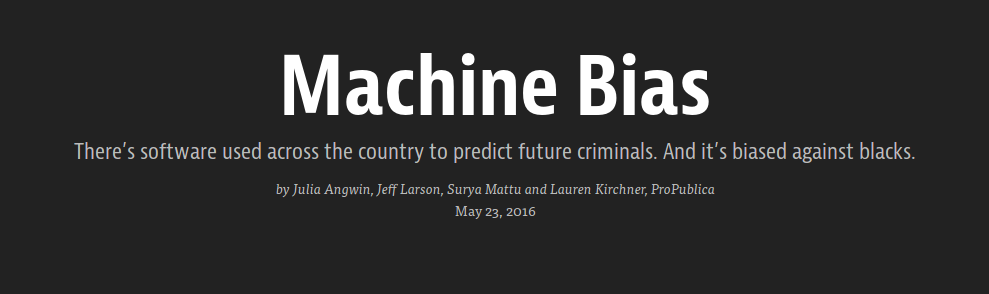## COMPAS: An observational debate

Probublica's main charge was observational.

Black defendants experienced higher false positive rate.

Northpointe's main defense was observational.

Scores satisfy precision parity.

A classifier $C$ cannot simultaneously achieve (a) precision parity, (b) true positive parity, and (c) false positive parity unless:

• $C=Y$ (the classifier is perfect), or
• $\mathbb{P}\{Y=1\mid A=0\}=\mathbb{P}\{Y=1\mid A=1\}$ (base rates are equal)

Due to Kleinberg, Mullainathan, Raghavan (2016), and Chouldechova (2016), although stated somewhat differently.

## Observational criteria have inherent limitations

There are two scenarios with identical joint distributions,
but completely different interpretations for fairness.

In particular, no observational definition
can distinguish the two scenarios.

Due to H, Price, Srebro (2016)

## Part III: Beyond Observational Measures

Causality

Deep dive into causal graphs, causal inference, interventions, matching

Develop causal fairness criteria (definitions, algorithms, trade-offs, ...)

Relationship to similarity-based fairness notions ("individual fairness")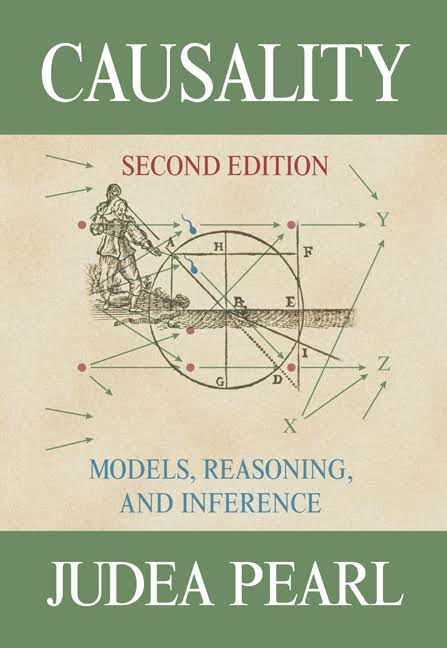## Part IV: Measurement and sampling

Measurement theory, sampling theory

Developing awareness of pitfalls

Understand data-generating processes better

## Part V: Legal and policy perspectives

Understand legal challenges technical work faces

Think through possibility of policy recommendations